International Journal of Materials Science and Applications
Volume 5, Issue 1, January 2016, Pages: 5-17

Gaseous Metal Hydrides MBeH3 and M2BeH4 (M = Li, Na): Quantum Chemical Study of Structure, Vibrational Spectra and Thermodynamic Properties

Awadhi Shomari*, Tatiana P. Pogrebnaya, Alexander M. Pogrebnoi

Department of Materials, Energy Science and Engineering, the Nelson Mandela African Institution of Science and Technology (NM–AIST), Arusha, Tanzania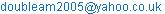(A. Shomari)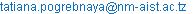(T. P. Pogrebnaya)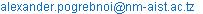(A. M. Pogrebnoi)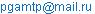(A. M. Pogrebnoi)

Awadhi Shomari, Tatiana P. Pogrebnaya, Alexander M. Pogrebnoi. Gaseous Metal Hydrides MBeH3 and M2BeH4 (M = Li, Na): Quantum Chemical Study of Structure, Vibrational Spectra and Thermodynamic Properties. International Journal of Materials Science and Applications. Vol. 5, No. 1, 2016, pp. 5-17. doi: 10.11648/j.ijmsa.20160501.12

Abstract: The theoretical study of complex hydrides MBeH3 and M2BeH4 (M = Li, Na) have been carried out using DFT MP2 methods with basis set 6-311++G (d, p). The optimized geometrical parameters, vibrational spectra and thermodynamic properties of the hydrides and subunits MH, M2H+, M2H2, BeH2, BeH3 have been determined. Two geometrical configurations, cyclic (C2v) and linear (Cv), were found for pentaatomic MBeH3 molecules, the cyclic isomer being predominant. Three isomers of M2BeH4 molecules were revealed of the following shapes: two-cycled (D2d), polyhedral (C2v) and hexagonal (C2v). Among these structures polyhedral isomer was found to have the lowest energy. The relative abundance of the M2BeH4 isomers in saturated vapour was analyzed. The enthalpies of formation ∆fH°(0) of complex hydrides in gaseous phase were determined (in kJ×mol-1): 105 ± 26 (LiBeH3), 63 ± 37 (Li2BeH4), 121 ± 27 (NaBeH3), and 117 ± 39 (Na2BeH4). The thermodynamic stability of the hydrides was examined through Gibbs free energies for heterophase decomposition.

Keywords: Complex Hydrides, Geometrical Structure, Vibrational Spectra, Enthalpy of Dissociation, Enthalpy of Formation, Heterophase Decomposition, Hydrogen Storage Materials

1. Introduction

Fossil fuels as the main source of energy worldwide are depleting, unsteady in terms of price, and have a negative impact to both the environment and humans beings . Hydrogen shows a promising interest as a synthetic fuel. In order to use hydrogen as the source of energy and replacement of fossil fuels; it has to overcome three technical challenges associated with the production, storage and use . Chater reported that the problem of hydrogen storage remains as the most challenging .

The large-scale deployment of vehicular fuel cells is hindered by the absence of a commercially feasible hydrogen storage technology. A selection of comparatively lightweight, low-cost, and high-capacity hydrogen storage devices must be available in a variety of sizes to meet different energy needs . The use of hydrogen as fuel in transport offers the greatest challenge towards system design. The criteria for a practical hydrogen store for mobile applications have been outlined by the U. S. Department of Energy .

Complex metal hydrides studied recently considered as promising materials for hydrogen storage . When Bogdanović and Schwickardi  announced the reversibility of the catalyzed sodium alanate NaAlH4 in hydrogen desorption and absorption reactions at ambient condition, several researches [8-12] have been focused on alkali complex hydrides particularly in the kinetics viewpoint. The complex hydrides Li2MH5 (M = B or Al) were previously studied theoretically and it was shown that these materials are stable at low temperatures and suggested to be potential for hydrogen storage purposes . The prediction and synthesis of hydride compound with sufficient amount of hydrogen contents were done in . A lithium–hydride bonding in complexes HMgHLiX with different ligands X including hydrogen was studied theoretically at MP2/6-311++G (d, p) level . Vajeeston  investigated the atomic arrangements, electronic structures, and bonding nature within the MMgH3 (M = Li, Na, K, Rb, Cs) series so as to determine the stability of these materials for hydrogen storage applications.

The decomposition of the complex metal hydrides such as the alkali metal tetrahydroborides to release hydrogen gas was reported in  to proceed in the following two channels:

MBH4 MH + BH3                 (1)

MBH4 MH + B + 3/2H2          (2)

Theoretical investigation of structural, electronic and thermodynamic properties of crystalline Na2BeH4 and the structural transition from α- to β-Na2BeH4 has been performed in .

This study aims at theoretical investigation of complex hydrides MBeH3 and M2BeH4 (M = Li, Na) in gaseous state implying a potential application for hydrogen storage. The content of hydrogen is 15.8% (LiBeH3), 8.6% (NaBeH3), 14.8% (Li2BeH4), and 6.8% (Na2BeH4). The targets are to determine the structure, geometrical parameters, vibrational spectra and thermodynamic properties of the complex hydrides and subunits they composed of and examine the thermodynamic stability of the hydrides with respect to different channels of decomposition. Therefore, our work will provide useful information on the structural and thermodynamic properties of the species and contribute to an exploration of the hydrides for hydrogen storage application.

2. Computational Details

The calculations were carried by implementing density functional theory (DFT) with hybrid functional B3PW91 , and second-order Møller–Plesset perturbation theory (MP2) with the basis set 6-311++G(d, p). In order to find out the accuracy of calculated results, the properties of the diatomic alkali metal hydride molecules were computed by using two different DFT hybrid functionals, B3P86 and B3PW91, and MP2 method together with the said basis set; the calculated properties were then compared with available experimental data. The optimization of geometrical parameters and vibrational spectra computations were performed using the PC GAMESS (General Atomic and Molecular Electronic Structure System) program , Firefly version 8.1.0 . Geometrical structures and IR spectra were visualized using the wxMcMolPlt  and ChemCraft software . The thermodynamic functions were determined in rigid rotator-harmonic oscillator approximation by using Open thermo software .

The enthalpies of dissociation reactions r(0) were computed using the formulae:

Δr(0) = ∆rE + ∆rε                 (3)

rε = 1/2hc(∑ωi prod – ∑ωi react       (4)

where rE is the energy of the reaction calculated through the total energies E of the species, rε is the zero point vibration energy (ZPVE) correction, ∑ωi prod and ∑ωi react are the sums of the vibration frequencies of the products and reactants respectively. The enthalpy of formation was computed by the underwritten equation:

rH°(0)= ∑∆fH°(0)­prod – ∑∆fH°(0)react         (5)

where ∑∆fH°(0)­prod and ∑∆fH°(0)react are enthalpies of formation of products and reactants, respectively. The values of ∑∆fH°(0)react were taken from Ivtanthermo Database . The thermodynamic stability of the complex hydrides was examined through Gibbs free energy rG°(T) of dissociation reactions. The values of rG°(T) were calculated by the formula:

rG°(T) = rH°(T) - TrS°(T)             (6)

where ΔrH°(T) and ΔrS°(T) are the enthalpy and entropy of the reaction at temperature T.

3. Results and Discussion

3.1. Subunits of Complex Hydrides

Diatomic molecules, NaH, LiH and H2. Two DFT hybrid functionals, B3P86 and B3PW91, together with MP2 were used to calculate molecular parameters: equilibrium internuclear distance, normal vibrational frequency, and dipole moment (Table 1). To test an accuracy of the calculated results a comparison with the available experimental data has been done. The calculated parameters do not contradict to the experimental values [26-29]. Among two DFT methods, B3PW91 and B3P86, the former provided a bit more accurate results. Thereby the results for other species considered are represented as found by DFT/B3PW91 and MP2 methods.

Table 1. Properties of diatomic molecules.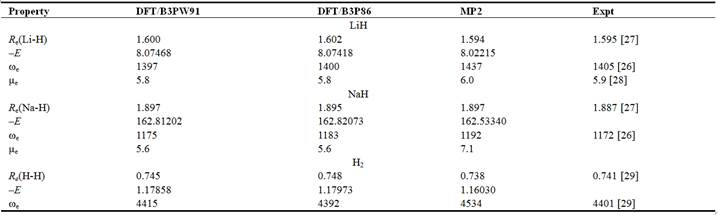Notes: here and hereafter in Tables 2–7, Re is the equilibrium internuclear distance in Å, E is the total energy in au, ωe is the vibrational frequency in cm–1, μe is the dipole moment in D.

Triatomic molecule BeH2 and ions M2H+ (M = Li, Na).

The characteristics of the BeH2 molecule are summarized in Table 2. The values obtained through the two methods are generally in agreement with each other and reference data . The values of equilibrium internuclear distance by DFT and MP2 are slightly shorter, by 0.007 Å and 0.014 Å, than the experimental value, while the valence asymmetric frequency ω2 is overrated by 3.2% (DFT) and 5.5% (MP2) respectively compared to experimental magnitude. The structure of the triatomic ions M2H+ is linear of Dh symmetry (Fig. 1 a); the results are displayed in Table 3. The experimental reference data are not available.

Table 2. Properties of triatomic molecule BeH2 (D∞h).Note: the parenthesized values near frequencies are the IR intensities in D2 amu–1 Å–2.

Table 3. Properties of triatomic ion, M2H+ (M= Li, Na), Dh symmetry.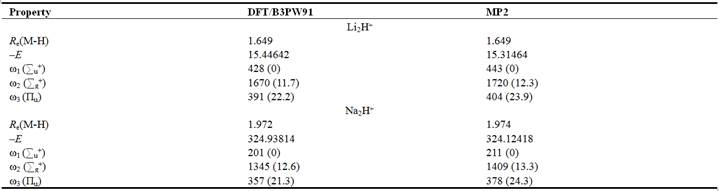The properties calculated follow the trend of that for the diatomic molecules MH (Table 1). It is worth mentioning that the internuclear distance Re(Li-H) in Li2H+ is longer by ~ 0.05 Å compared to that in LiH and Re(Na-H) in Na2H+ is longer by ~0.08 Å than that in NaH. The vibration frequencies calculated by two methods are in a good agreement between each other, respectively.

Tetraatomic ion BeH3 and molecules M2H2 (M= Li, Na).

The properties of the tetraatomic species are displayed in Tables 4 and 5; their structures are shown in Figs. 1 b, c. The tetraatomic ion BeH3 has the planar equilibrium configuration of the D3h symmetry. The values obtained through two methods are generally in agreement with each other. For the M2H2 molecules, the values of internuclear distances, valence angles, and vibrational frequencies calculated by DFT and MP2 fairly match with each other respectively and with the theoretical results obtained previously . The calculated enthalpy of dimerization for Li2H2 is in agreement within uncertainty limit with the experimental magnitude by Wu et al. .

Table 4. Properties of tetraatomic BeH3 ion, D3h symmetry.Table 5. Properties of dimers M2H2 (M=Na, Li), D2h symmetry.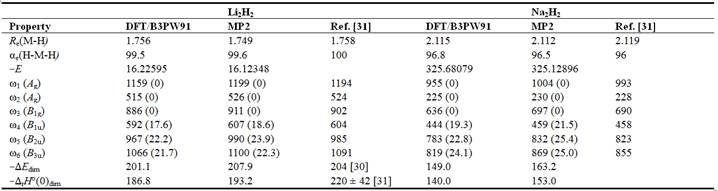Note: Here and hereafter αe is bond angle in degrees; the values Edim and ∆rH°(0)dim are the energies and enthalpies of dimerization reactions 2MH = M2H2 in kJ mol–1.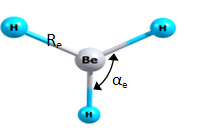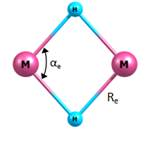(a)                                 (b)                             (c)

Figure 1. Equilibrium geometrical structure of the species: (a) M2H+D¥h; (b) dimers M2H2, D2h; (c) BeH3-, D3h.

3.2. Geometrical Structure and Vibrational Spectra of Pentaatomic Molecules LiBeH3 and NaBeH3

Two possible geometrical configurations, cyclic (C2v) and linear (Cv), were considered for pentaatomic MBeH3 molecules (Fig. 2). The calculated equilibrium geometrical parameters and vibrational frequencies for cyclic isomer are shown in Table 6. The binding in the cyclic isomer may be considered through an attachment of M+ cation to BeH3 anion. Within the MBeH3 molecules, the fragment BeH3 is distorted compared to free BeH3 anion. In the latter the bond lengths and angles are equivalent, Re(Be-H) ≈ 1,42 Å (Table 4) while in the MBeH3 molecules the bridge distances Re(Be-H) are elongated to 1.44-1.45 Å; and the terminal distance is shortened to ~1.35 Å; the bond angles become also non-equivalent, the angle βe(H4-Be1-H5) decreases to 104° in LiBeH3 and 110° in NaBeH3. Thus the BeH3 moiety looks alike in both LiBeH3 and NaBeH3 molecules.

The IR spectra of MBeH3 (C2v) molecules are presented in Fig. 3. The similarity of the vibrational bands is observed for LiBeH3 and NaBeH3. For instance the most intensive bands correspond to the Be-H stretching vibrations at 1528 cm–1 (LiBeH3) and 1555 cm–1 (NaBeH3). The highest vibration frequencies correspond to the Be1-H3 stretching vibrations at 2029 cm–1 (LiBeH3) and 1980 cm–1 (NaBeH3). The bending vibration H-Be-H is observed at 849 cm–1 (LiBeH3) and 833 cm–1 (NaBeH3).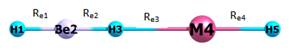(a)                                                       (b)

Figure 2. Equilibrium geometrical structure of MBeH3 (M = Li, Na) molecules: (a) cyclic, C2v; (b) linear, Cv.

Table 6. Properties of MBeH3 (M= Li, Na) molecules (C2v).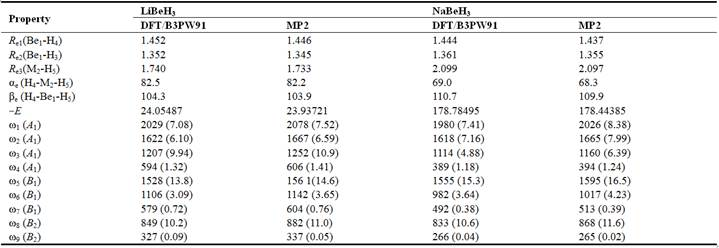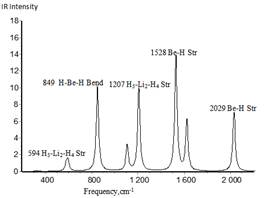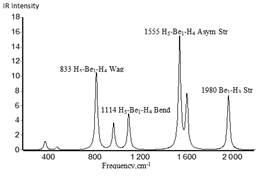(a)                                   (b)

Figure 3. IR spectra of MBeH3 (M = Li, Na) molecules, C2v isomer, calculated by DFT/B3PW91: (a) LiBeH3 (b) NaBeH3.

Table 7. Properties of MBeH3 (M= Li, Na) molecules (Cv).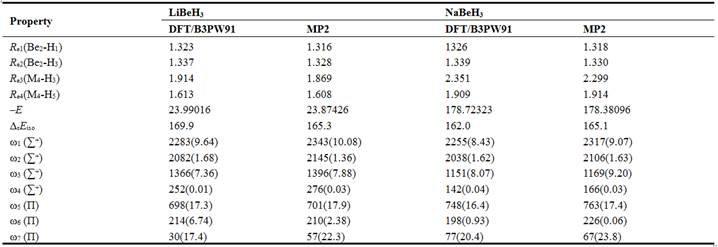Note: ΔrEiso is the relative energy of linear isomer regarding cyclic, ΔrEiso = ElinEcycl, in kJ mol–1.

The properties of the MBeH3 molecules of linear configuration are shown in Table 7. The linear isomer MBeH3 may be represented through linkage of the MH and BeH2 molecules. Worth to mention that for lithium bonding complexes HMgH···LiH the linear structure was considered in , while existence of possible isomers had not been taken into account. Our results for MBeH3 show that the energy of the linear isomer appeared to be much higher compared to the cyclic one, i. e. by 165 kJ mol–1 (MP2, both for LiBeH3 and NaBeH3). It is also worth to note a low frequency of vibration ω7 which corresponds to bending of the Li-H-Be fragment. Moreover when the parameters of the linear isomers were calculated using DFT/P3P86 method the imaginary frequency for LiBeH3 was revealed which indicates low stability of the linear structure with respect to bending deformation. Hence only this cyclic isomer was considered further in examination of thermodynamic properties of MBeH3 hydrides.

3.3. Geometrical Structure and Vibrational Spectra of Heptaatomic Li2BeH4 and Na2BeH4 Molecules

Several different geometrical shapes of the M2BeH4 molecules have been considered: bipyramidal one with a tail of C2v symmetry; polyhedral (compact or hat-shaped), C2v; two-cycles, D2d; and hexagonal shape, C2v. Among these four configurations the first one was found to be unstable as imaginary vibrational frequencies were revealed. The rest three structures were proved to correspond to the minima at the potential energy surface and therefore appeared to be isomers of M2BeH4 molecules. Hereafter these isomers are denoted as I, II, and III, for C2v compact, D2d, and C2v hexagonal, respectively; the equilibrium geometrical configurations are shown in Fig. 4 and the parameters are displayed in Tables 8-10.

The binding in the polyhedral and two-cycled structures may be considered through an attachment of two M atoms to a slightly distorted tetrahedral BeH4 moiety. In the first isomer there are two types of Be-H bonds with internuclear separations Re1(Be-H) ≈ 1.53 Å and Re2(Be-H) ≈ 1.42 Å; the averaged of these two values, 1.47 Å, is very close to the distance Re(Be-H) in the D2d isomer. The averaged valence angle H-Be-H in BeH4 fragment of the polyhedral isomers is about 109° that is almost equal to the tetrahedral angle. In the D2d isomer, the angle H-Be-H is 104° (Li2BeH4) and 110° (Na2BeH4) that is also close to the tetrahedral angle. The hexagonal molecule may be considered through a combination of BeH3 and M2H+ subunits. The geometrical parameters, the bridge Be-H bond lengths (~1.42 Å) and valence angle βe(H-Be-H) ≈ 117°, of the hexagonal M2BeH4 molecules are similar to the respective parameters in free BeH3 ion as well as in cyclic MBeH3 molecules.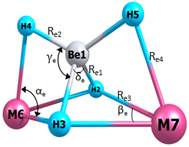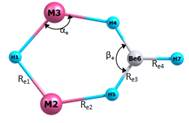(a)                                 (b)                              (c)

Figure 4. Equilibrium geometrical configurations of M2BeH4 isomers: (a) I, polyhedral (C2v); (b) II, two-cycled (D2d); (c) III, hexagonal (C2v).

Table 8. Properties of M2BeH4 (M = Li, Na) molecules (C2v, compact structure).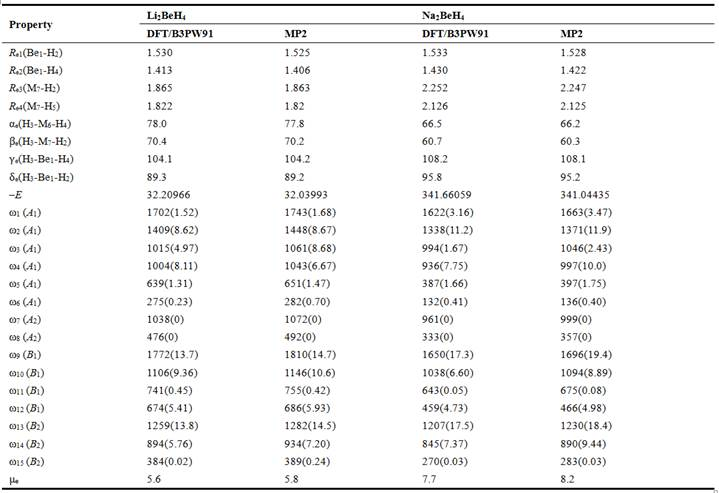Table 9. Properties of M2BeH4 (M = Li, Na) molecules (D2d).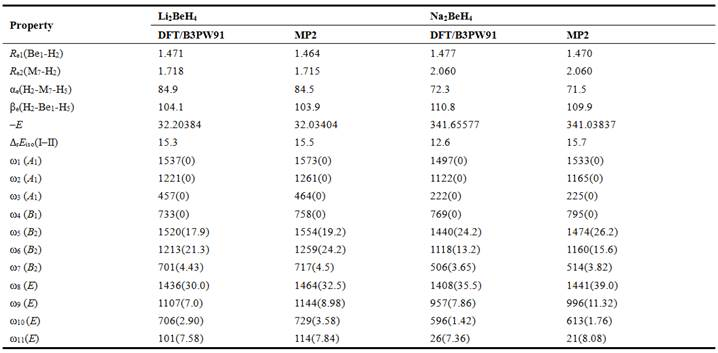Note: rEiso(I-II) is the energy of isomerization reaction M2BeH4 (I, C2v, comp)= M2BeH4 (II, D2d), rEiso(I-II) = E(II) E(I), in kJ×mol-1.

The IR spectra of three isomers of Li2BeH4 and Na2BeH4 molecules are presented in Fig. 5. By comparing IR spectra of Li2BeH4 and Na2BeH4 molecules, alike features may be observed for the isomers of the same symmetry. For the polyhedral isomer I, the bands of high intensity at 1259 cm–1, 1772 cm–1 (Li2BeH4) and 1207 cm–1, and 1650 cm–1 (Na2BeH4) correspond to H-Be-H asymmetrical stretching vibrations of BeH4 moiety. For the two-cycled D2d isomer, similar H-Be-H asymmetrical stretching vibrations of BeH4 moiety are observed at 1436 cm–1, 1520 cm–1 (Li2BeH4) and 1408 cm–1, 1440 cm–1 (Na2BeH4). The most intensive band in spectrum of Li2BeH4 D2d is seen at 1213 cm–1 and corresponds to the H-Li-H asymmetrical stretching mode, in Na2BeH4 D2d the similar vibration is observed at 1118 cm–1. For the hexagonal isomer the most intense bands appear at 1764 cm–1 (Li2BeH4) and 1742 cm–1 (Na2BeH4) and are characterized by stretching modes of the BeH3 fragment. Other similarities may be noted between two hexagonal species as wagging vibrations at 444 cm–1 Li-H-Li, 794 cm–1 H-Be-H (Li2BeH4) and 344 cm–1 Na-H-Na, 797 cm–1 H-Be-H (Na2BeH4). The vibration of highest frequency at about 2000 cm–1, both for Li2BeH4 and Na2BeH4, corresponds to the terminal bond Be-H stretching mode, that is the highest frequency correlates with the shortest bond length Re(Be-H) = 1.36 Å.

Table 10. Properties of M2BeH4 (M = Li, Na) molecules (C2v, hexagonal structure).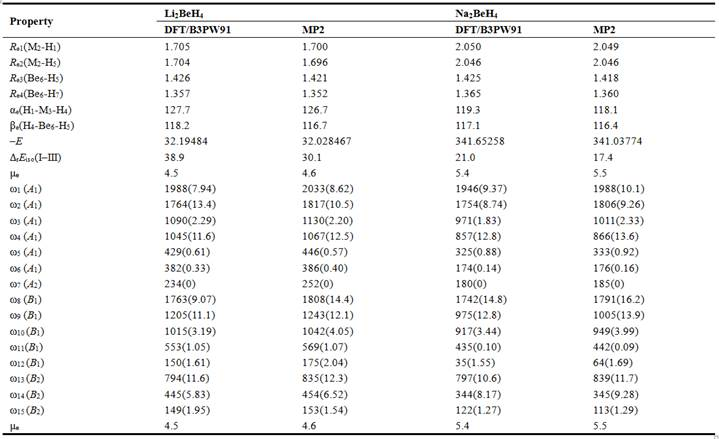Note: rEiso(IIII) is the energy of isomerization reaction M2BeH4 (I, C2v, comp) = M2BeH4 (III, C2v, hex), rEiso(IIII) = E(III) E(I), in kJ×mol-1.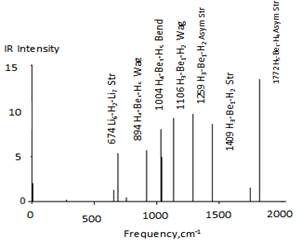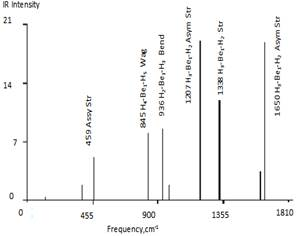(a)                                                    (b)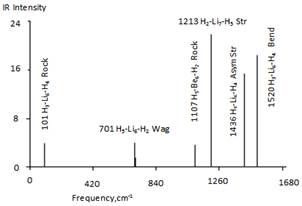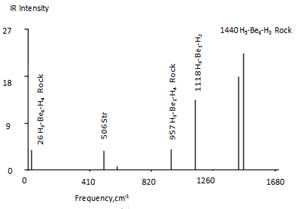(c)                                                    (d)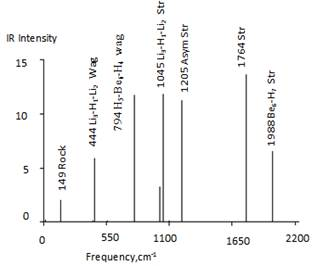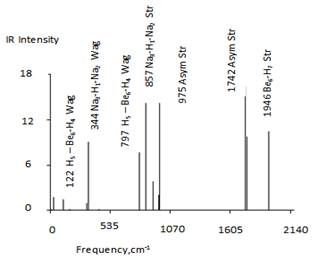(e)                                                    (f)

Figure 5. IR spectra of complex hydrides M2BeH4 (M = Li, Na) calculated by DFT/B3PW91: (a) Li2BeH4 (C2v, compact); (b) Na2BeH4 (C2v, compact); (c) Li2BeH4 (D2d); (d) Na2BeH4(D2d); (e) Li2BeH4 (C2v, hexagonal); (f) Na2BeH4 (C2v, hexagonal).

The relative energies ∆rEiso of the isomers II and III regarding I given in Tables 9, 10 were calculated for the following isomerisation reactions:

M2BeH4(I, C2v, comp) = M2BeH4(II, D2d       (7)

M2BeH4(I, C2v, comp) = M2BeH4(III, C2v, hex).       (8)

The values of ΔrEiso are positive: for reaction R1 15.5 kJ mol-1 (Li2BeH4), 15.7 kJ mol-1 (Na2BeH4), and for R2 30.1 kJ mol-1 (Li2BeH4), 17.4 kJ mol-1 (Na2BeH4) according to MP2 calculations. Therefore among three isomers, I, II, and III, the first one has the lowest energy, followed by D2d, and the hexagonal: E(I) < E(II) < E(III) for both molecules. The energy difference between isomers II and III is 14.6 kJ mol-1 (Li2BeH4), and 1.7 kJ mol-1 (Na2BeH4) in favour of D2d, thus worth to note the isomers II and III of the Na2BeH4 molecule are comparable by energy.

To evaluate the relative concentration of the isomers in the equilibrium vapour, the thermodynamic approach was applied. The following equation was used: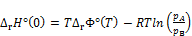(9)

where ∆rH°(0) is the enthalpy of isomerisation of the reaction; T is absolute temperature; ∆rΦ°(T) is the reduced Gibbs energy of the reaction, Φ°(T) = -[H°(T)-H°(0)-TS°(T)]/T; pA/pB is the pressure ratio between two isomers, that is pII/pI for reaction R1 and pIII/pI for R2. The values of ∆rH°(0) were calculated using isomerization energies ∆rEiso and the ZPVE corrections ∆rε by Eqs. (3) and (4). The relative concentrations pA/pB have been calculated for the temperature range between 500 and 2000 K; the plots are shown in Fig. 6. The graphs show that the relative concentrations of the isomers II and III increase with temperature increase for both molecules, for Li2BeH4 the growth is slow compared to Na2BeH4. At 1000 K for Li2BeH4 and Na2BeH4 the values of pII/pI are equal to 0.6 and 5.2, respectively, while the ratios pIII/pI are 2.5 and 30, respectively. Therefore the isomer I of Li2BeH4 molecule is more abundant at moderate temperatures, but at higher temperatures its concentration is noticably decreasing. For Na2BeH4 the hexagonal isomer is much more abundant compared to either isomers I and II. The fraction of each isomer of Na2BeH4 was estimated as xi = pi/(pI + pII + pIII) where i stands for I, II, or III, the results for two selected temperatures are given in Table 11. Thus as seen the hexagonal isomer of Na2BeH4 is predominant in a broad temperature range and its concentration is increasing with temperature raise.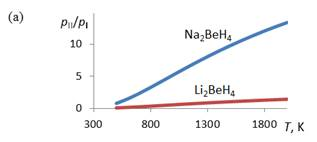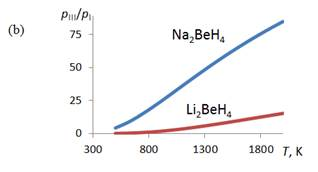Figure 6. Relative abundance pA/pB versus temperature for three isomers of complex hydrides M2BeH4 by MP2 method: (a) pII(D2d)/pI(C2v, comp); (b) pIII(C2v, hex)/ pI(C2v, comp).

Table 11. The fraction xi of isomers I, II, and III in equilibrium vapour of Na2BeH4.3.4. Thermodynamic Properties of Complex Hydrides

3.4.1. The Enthalpies of Dissociation Reactions and Enthalpies of Formation of Molecules

Different dissociation reactions of the complex hydrides MBeH3 and M2BeH4 have been examined; for the latter the polyhedral isomer of C2v symmetry was considered as lowest by energy. The calculated energies and enthalpies of gas-phase reactions are represented in Tables 9 and 10; the results obtained by DFT/B3PW91 and MP2 methods. Two types of dissociation reactions of complex hydrides, MBeH3 and M2BeH4 were considered: a partial dissociation and complete reduction of the hydride with hydrogen gas release. The values of ∆rH°(0) show that all reactions proceed with the absorption of energy (endothermic). The partial dissociation of both penta- and heptaatomic hydrides requires much less energy than reaction with hydrogen formation. The most energy consuming reactions are those with H2 evolving (reactions 2 for MBeH3 and 6, 7 for M2BeH4) and dissociation into ionic subunits M2H+ and BeH3 (reactions 8).

The enthalpies of formation ∆fH°(0) of the complex hydrides were calculated through the enthalpies of the reactions and enthalpies of formation of the gaseous products, Li, Na, H2, LiH, NaH  and BeH2 . The enthalpies of formation of M2H2 molecules involved in reactions 5 were obtained through the enthalpies of dimerization reactions (Table 5), the averaged values of ∆fH°(0) between DFT/B3PW91 and MP2 methods were accepted: 90 ± 10 kJ×mol-1 (Li2H2) and 139 ± 10 kJ×mol-1 (Na2H2). The enthalpies of formation of the penta- and heptaatomic hydrides are presented in the far right column in Tables 12, 13. The enthalpies of formation of MBeH3 molecules are accepted as the averaged values found through the enthalpies of reactions 1 and 2; similarly for M2BeH4 through reactions 3-7. Uncertainties were estimated as half-differences between maximum and minimum magnitudes. The accepted values of ∆fH°(0) are gathered in Table 14.

Table 12. The energies and enthalpies of gas-phase dissociation reactions, and enthalpies of formation of gaseous complex hydrides LiBeH3 and Li2BeH4; all values are given in kJ mol-1.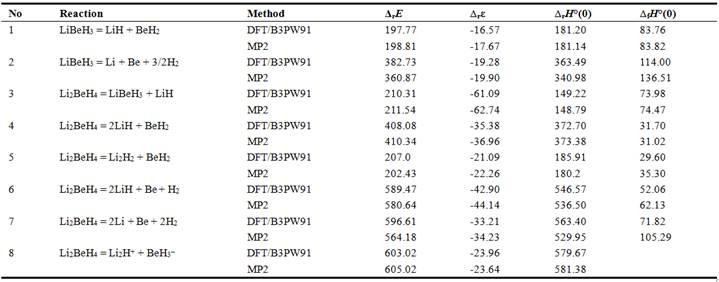Table 13. The energies and enthalpies of gas-phase dissociation reactions, and enthalpies of formation of gaseous complex hydrides NaBeH3 and Na2BeH4; all values are given in kJ mol-1.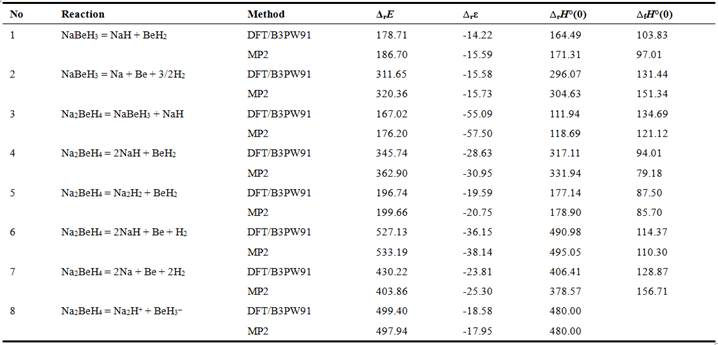Table 14. Accepted enthalpies of formation (in kJ mol1) of gaseous complex hydrides MBeH3 and M2BeH4 (M = Li, Na).Stability of the gaseous complex hydrides MBeH3 and M2BeH4 regarding heterophase decomposition with hydrogen release were also considered. The enthalpies of the heterophase reactions were calculated and given in Table 12. The required enthalpies of formation of Be, LiH, NaH, Li, Na in condensed phase were taken from . In the heterophase reactions considered, beryllium is in solid state, the alkali metal hydrides are in gas-phase (reactions 1, 4) or in condensed phase (reactions 2, 5); complete decomposition is described by reactions 3, 6. The results show that the reactions in which gaseous MH are among the products are endothermic, while the rest reactions are exothermic; the biggest energy being released in reactions 2 and 5 with MH(c).

3.4.2. Thermal Stability of the Complex Hydrides and Thermodynamic Favourability of the Reactions

The thermodynamic stability of the complex hydrides MBeH3 and M2BeH4 was examined through Gibbs free energies for heterophase reactions shown in Table 15. The temperature dependences of ΔrG° are presented in Figs. 7-9. For the reactions in which MH is in gaseous phase ΔrG° are negative at moderate and elevated temperatures (Fig. 7); the decomposition reactions are thermodynamically favoured at temperatures above 350 K (NaBeH3), 500 K (LiBeH3), 800 K (Na2BeH4) and 1000 K (Li2BeH4). Thus the MBeH3 hydrides appeared to be less stable thermodynamically than M2BeH4 and Na-containing hydrides are less stable compared to Li-hydrides.

For reactions in which both MH and Be are in condensed phase (Fig. 8) the values of ΔrG°(T) are negative for whole temperature range considered. The Na-containing hydrides are slightly more stable than Li-hydrides as the values of ΔrG°(T) for the former are less negative. The inflections on the curves correspond to phase change transition of the products, namely the melting points of LiH(c) and NaH(c) at 965 K and 911 K , respectively. The Gibbs free energy for the decomposition reaction of MBeH3 decreases with temperature raise, while for M2BeH4 hydrides the values of ΔrG° pass through maximum at temperatures of the phase transitions. Here the entropy has an impact on ΔrG°(T): as a jump of entropy at phase transition of MH(c) occurs hence the contribution of entropy factor TΔrS increases with temperature raise.

Table 15. The enthalpies of heterophase dissociation reactions of gaseous hydrides MBeH3 and M2BeH4.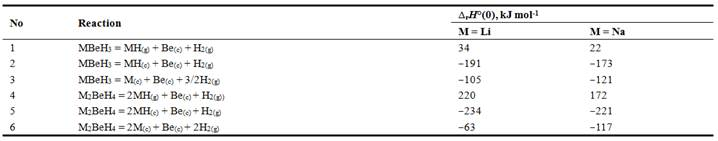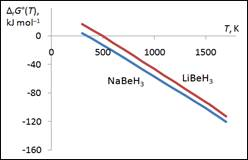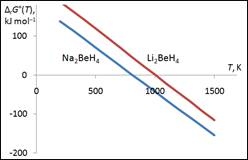(a)                                                    (b)

Figure 7. Gibbs free energy ΔrG°(T) against temperature for heterophase decomposition reactions of complex hydrides MBeH3 and M2BeH4: (a) MBeH3(g) = MH(g) + Be(c) + H2(g); (b) M2BeH4(g) = 2MH(g) + Be(c) + H2(g).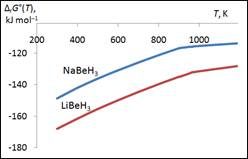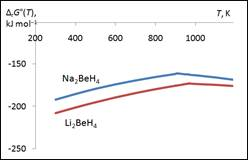(a)                                                      (b)

Figure 8. Gibbs free energy ΔrG°(T) against temperature for heterophase decomposition reactions of complex hydrides MBeH3 and M2BeH4: (a) MBeH3(g) = MH(c) + Be(c) + H2(g); (b) M2BeH4(g) = 2MH(c) + Be(c) + H2(g).

For the reactions with complete dissociation into alkaline metal and beryllium in condensed phase (Fig. 9) the Gibbs free energies are negative in the temperature range considered and decreasing with temperature increase, this indicates that the decomposition processes are spontaneous. In contrast to previous case (Fig. 8), the Na-containing hydrides are less stable than Li-hydrides as the values of ΔrG°(T) are less negative for the latter.

For different channels of dissociation of the hydrides, a correlation between ΔrG°(T) and ΔrH°(0) values may be noted: the lower is the enthalpy of the reaction the more negative are ΔrG°(T) and hence the more favourable the decomposition process. For instance, for the reactions with gaseous alkali hydrides MH the enthalpies ∆rH°(0) are positive, ~20–30 kJ mol–1 (MBeH3) and ~170–220 kJ mol–1 (M2BeH4); then the Gibbs free energies are positive at low and moderate temperatures and turn negative at certain temperatures said (Fig. 7). This implies that the reversibility of the reactions is able to be attained. For other heterogeneous reactions the ∆rH°(0) values are negative, the Gibbs free energies are negative (Figs. 8, 9) that is the decomposition of the hydrides, both MBeH3 and M2BeH4, is spontaneous in the whole temperature range considered. The reaction with Li/Na and Be in condensed phase the reversibility may be achieved by pressure increase (Le Châtelier's principle). The reversibility of the decomposition reactions of hydrides is one of the requirements for hydrogen storage materials.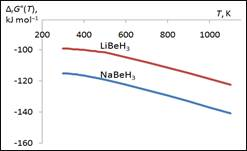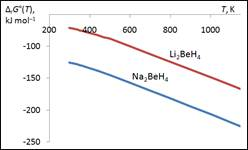(a)                                          (b)

Figure 9. Gibbs free energy ΔrG°(T) against temperature for heterophase decomposition reactions of complex hydrides MBeH3 and M2BeH4: (a) MBeH3(g) = M(c) + Be(c) + 3/2H2(g); (b) M2BeH4(g) = 2M(c) + Be(c) + 2H2(g).

4. Conclusion

The geometrical parameters, vibrational spectra and thermodynamic properties of the complex hydrides MBeH3 and M2BeH4 (M = Li, Na) and subunits have been determined using DFT/B3PW91 and MP2 methods. The results obtained by both methods are in a good agreement between each other and with the reference data available for subunits MH, BeH2, M2H2. The enthalpies of different gas-phase dissociation reactions were computed; the enthalpies of formation of the complex hydrides were found. The Gibbs free energies ΔrG°(T) of heterophase decomposition of MBeH3 and M2BeH4 with hydrogen release were analyzed. It was shown the reactions the products of which were gaseous alkaline metals and solid beryllium may be reversible at moderate temperatures. The reactions of complete decomposition (products are M(c), Be(c), H2) were shown to be spontaneous at a broad temperature range; the reversibility of the reactions may be attained if certain conditions are provided. The complex hydrides MBeH3 and M2BeH4 (M = Na or Li) may be considered as promising candidates for hydrogen storage applications as they showed the feasibility of hydrogen gas production.

Authors’ Contributions

Authors participated equally in all steps to the completion of this work.

Acknowledgment

The authors are very thankful to the government of Tanzania through The Nelson Mandela African Institution of Science and Technology for supporting and sponsoring this study.

References

1. Patlitzianas, K. D., Doukas, H., Kagiannas, A. G., and Psarras, J., Sustainable energy policy indicators: Review and recommendations. Renewable Energy, 2008. 33(5): p. 966-973.
2. Sartbaeva, A., Kuznetsov, V., Wells, S., and Edwards, P.,Energy Environ. Sci. 2008, 1, 79 Armaroli, N., Balzani, V. ChemSusChem, 2011. 4: p. 21.
3. Chater, P.A., Mixed anion complex hydrides for hydrogen storage. 2010, University of Birmingham.
4. Alapati, S.V., Johnson, J. K., and Sholl, D. S., Identification of destabilized metal hydrides for hydrogen storage using first principles calculations. J. Phys. Chem. B, 2006. 110(17): p. 8769-8776.
5. Ahluwalia, R., Hua, T., and Peng, J., On-board and off-board performance of hydrogen storage options for light-duty vehicles. Int. J. Hydrogen Energy, 2012. 37(3): p. 2891-2910.
6. Jena, P., Superhalogens: A Bridge between Complex Metal Hydrides and Li Ion Batteries. J. Phys. Chem. Lett., 2015. 6(7): p. 1119-1125.
7. Bogdanović, B., and Schwickardi, M., Ti-doped alkali metal aluminium hydrides as potential novel reversible hydrogen storage materials. J.Alloys Compd., 1997. 253: p. 1-9.
8. Zaluska, A., Zaluski, L., and Ström–Olsen,J., Nanocrystalline magnesium for hydrogen storage. J.Alloys Compd., 1999. 288(1): p. 217-225.
9. Zidan, R. A., Takara S., Hee, A. G., and Jensen, C. M., Hydrogen cycling behavior of zirconium and titanium–zirconium-doped sodium aluminum hydride. J. Alloys Compd., 1999. 285(1): p. 119-122.
10. Fletcher, G., Schmidt, M., and Gordon, M., Developments in parallel electronic structure theory. Adv. Chem. Phys., 1999. 110: p. 267-294.
11. Sandrock, G., Gross, K.., and Thomas, G., Effect of Ti-catalyst content on the reversible hydrogen storage properties of the sodium alanates. J.Alloys Compd., 2002. 339(1): p. 299-308.
12. Morioka, H., Kakizaki, K., Chung, S. C., and Yamada, A.,Reversible hydrogen decomposition of KAlH4. J. Alloys Compd., 2003. 353(1): p. 310-314.
13. Melkizedeck Hiiti Tsere, Tatiana P. Pogrebnaya, Alexander M. Pogrebnoi. Complex Hydrides Li2MH5 (M = B, Al) for Hydrogen Storage Application: Theoretical Study of Structure, Vibrational Spectra and Thermodynamic Properties. International Journal of Computational and Theoretical Chemistry.Vol. 3, No. 6, 2015, pp. 58-67. doi: 10.11648/j.ijctc.20150306.13.
14. Nakamori, Y., Miwa, K., Ninomiya, A., Li, H., Ohba, N., Towata S. I., Züttel, A. and Orimo, S. I.,Correlation between thermodynamical stabilities of metal borohydrides and cation electronegativites: First-principles calculations and experiments. Phys. Rev. B, 2006. 74(4): p. 045126.
15. Li, Q., Wang, Y., Li, W., Cheng, J., Gong, B., and Sun, J.,Prediction and characterization of the HMgHLiX (X= H, OH, F, CCH, CN, and NC) complexes: a lithium–hydride lithium bond. Phys. Chem. Chem. Phys., 2009. 11(14): p. 2402-2407.
16. Vajeeston, P., Ravindran, P., Kjekshus, A., and Fjellvåg, H.,First-principles investigations of the MMgH3 (M= Li, Na, K, Rb, Cs) series. J. Alloys Compd., 2008. 450(1): p. 327-337.
17. Züttel, A., Wenger, P., Rentsch, S., Sudan, P., Mauron, P., and Emmenegger, C., LiBH4 a new hydrogen storage material. J. Power Sources, 2003. 118(1): p. 1-7.
18. Hu, C., Wang, Y., Chen, D., Xu, D., and Yang, K., First principles calculations of structural, electronic and thermodynamic properties of Na2BeH4. Physical Review B, 2007. 76(14): p 144104.
19. Becke, A.D., Densityfunctional thermochemistry. III. The role of exact exchange. J. Phys. Chem., 1993. 98(7): p. 5648-5652.
20. Schmidt, M.W., Baldridge, K. K., Boatz, J. A., Elbert, S. T., Gordon, M. S., Jensen, J. H., Koseki, S., Matsunaga, K. A., Nguyen, K. A. and Su, S. General atomic and molecular electronic structure system. J. Comput. Chem., 1993. 14(11): p. 1347-1363.
21. Granovsky, A. A., Firefly version 8.1.0, www http://classic.chem.msu.su/gran/firefly/index.html.
22. Bode, B.M. and Gordon M. S., MacMolPlt: a graphical user interface for GAMESS. J. Mol. Graphics Modell., 1998. 16(3): p. 133-138.
23. Zhurko, G. and Zhurko, D., Chemcraft, Version 1.7 (build 365). 2013.
24. Tokarev, K. L., "OpenThermo", v.1.0 Beta 1 (C) ed., 2007-2009. http://openthermo.software.informer.com/.
25. Gurvich, L. V., Yungman, V. S., Bergman, G. A., Veitz, I. V., Gusarov, A. V., Iorish V. S., Leonidov, V. Y., Medvedev, V. A., Belov, G. V., Aristova, N. M., Gorokhov, L. N., Dorofeeva, O. V., Ezhov, Y. S., Efimov, M.E., Krivosheya, N. S., Nazarenko, I.I, Osina, E. L., Ryabova, V. G., Tolmach, P. I., Chandamirova, N. E., Shenyavskaya, E.A., "Thermodynamic Properties of individual Substances. Ivtanthermo for Windows Database on Thermodynamic Properties of Individual Substances and Thermodynamic Modeling Software", Version 3.0 (GlushkoThermocenter of RAS, Moscow, 1992-2000).
26. Irikura, K. K.,"Experimental Vibrational Zero-Point Energies: Diatomic Molecules" J. Phys. Chem. Ref. Data 36(2), 389, 2007.
27. NIST Diatomic Spectral Database (www.physics.nist.gov/PhysRefData/MolSpec/Diatomic/index.html).
28. NIST Chemistry Webbook (http://webbook.nist.gov/chemistry).
29. Huber, K.P.; Herzberg, G., Molecular Spectra and Molecular Structure. IV. Constants of Diatomic Molecules Van Nostrand Reinhold Co., 1979.
30. Jacox, M. E., Vibrational and Electronic Energy Levels of Polyatomic Transient Molecules, J. Phys. Chem.Ref. Data, Monograph 3 (1994) (updated data in NIST Chemistry Webbook - http://webbook.nist.gov/chemistry/.
31. Chen, Y. L., Huang C. H., and Hu, W. P., Theoretical study on the small clusters of LiH, NaH, BeH2, and MgH2. J. Phys. Chem. A, 2005. 109(42): p. 9627-9636.
32. Wu, C. and Ihle, H., Thermochemistry of the Dimer Lithium Hydride Molecule Li2H2 (g). 1982.

 Contents 1. 2. 3. 3.1. 3.2. 3.3. 3.4. 4.
Article ToolsAbstractPDF(1802K)